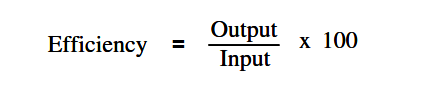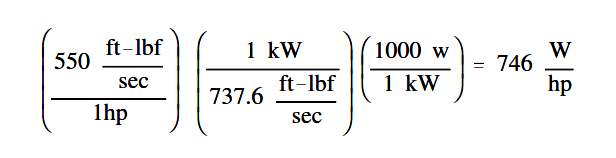# Losses in AC Generator

The load current flows through the armature in all AC generators. Like any coil, the armature has some amount of resistance and inductive reactance. The combination of these make up what is known as the internal resistance, which causes a loss in an AC generator.

When the load current flows, a voltage drop is developed across the internal resistance. This voltage drop subtracts from the output voltage and, therefore, represents generated voltage and power that is lost and not available to the load.

The voltage drop in an AC generator can be found using below Equation.

Voltage drop = IaRa + IaXLa

where

Ia = armature current
R= armature resistance
XLa = armature inductive reactance

#### Hysteresis Losses

Hysteresis losses occur when iron cores in an AC generator are subject to effects from a magnetic field. The magnetic domains of the cores are held in alignment with the field in varying numbers, dependent upon field strength. The magnetic domains rotate, with respect to the domains not held in alignment, one complete turn during each rotation of the rotor. This rotation of magnetic domains in the iron causes friction and heat. The heat produced by this friction is called magnetic hysteresis loss.

To reduce hysteresis losses, most AC armatures are constructed of heat-treated silicon steel, which has an inherently low hysteresis loss. After the heat-treated silicon steel is formed to the desired shape, the laminations are heated to a dull red and then allowed to cool. This process, known as annealing, reduces hysteresis losses to a very low value.

#### Mechanical Losses

Rotational or mechanical losses can be caused by bearing friction, brush friction on the commutator, and air friction (called windage), which is caused by the air turbulence due to armature rotation. Careful maintenance can be instrumental in keeping bearing friction to a minimum.

Clean bearings and proper lubrication are essential to the reduction of bearing friction. Brush friction is reduced by ensuring: proper brush seating, proper brush use, and maintenance of proper brush tension. A smooth and clean commutator also aids in the reduction of brush friction. In very large generators, hydrogen is used within the generator for cooling; hydrogen, being less dense than air, causes less windage losses than air.

#### Efficiency

Efficiency of an AC generator is the ratio of the useful power output to the total power input. Because any mechanical process experiences some losses, no AC generators can be 100 percent efficient. Efficiency of an AC generator can be calculated using below Equation.Example:

Given a 5 hp motor acting as the prime mover of a generator that has a load demand of 2 kW, what is the efficiency of the generator?

Solution:

In order to calculate efficiency, the input and output power must be in the same units. As described in Thermodynamics, the horsepower and the watt are equivalent units of power.

Therefore, the equivalence of these units is expressed with a conversion factor as follows.Input Power = 5 hp x 746 w/hp

Input Power = 3730 W

Output Power = 2 kW = 2000 W

Efficiency = output/input

Efficiency = 2000 W / 3730 W

Efficiency = 0.54 x 100%

Efficiency = 54%